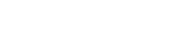# math.ceil

`FLOATmath.ceilFLOATx`

Available inall subroutines.

Computes the smallest integer value greater than or equal to the given value. In other words, round `x` towards positive infinity.

For example, 2.2, 2.5, and 2.7 all ceil to 3.0.

## Return Value

If `x` is `math.NAN`, a NaN will be returned.

If `x` is integral, ±0, `x` itself is returned.

If `x` is `math.POS_INFINITY` or `math.NEG_INFINITY`, an infinity of the same sign is returned.

Otherwise, the rounded value of `x` is returned.

BETA